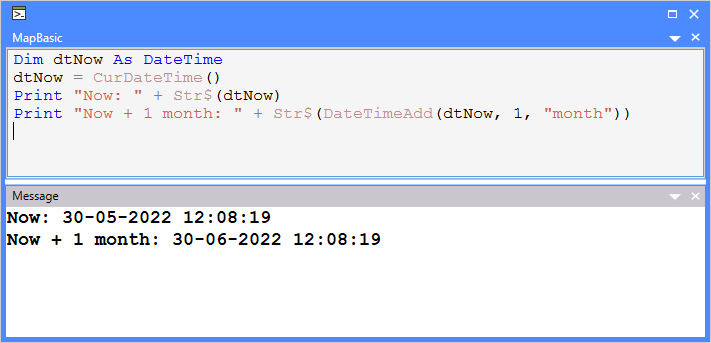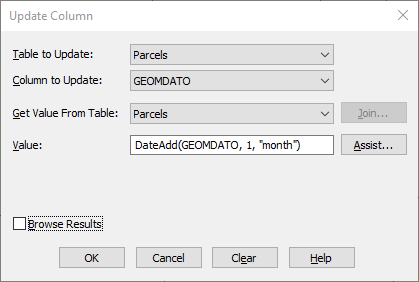# MapInfo Pro Developers User Group

View Only

## MapBasic Monday: Function for Adding a Value in a Specific Date Units

• #### 1.  MapBasic Monday: Function for Adding a Value in a Specific Date Units

Posted 27 days ago
Edited by Peter Møller 27 days ago
Happy #MapInfoMonday and #MapBasicMonday!

Today we will look at two functions that can help you add and subtract values to Date and DateTime values.

### What's the problem?

This might be your initial question, right? You can already add values to a Date and DateTime value.

Let's see how that works. I'll use a basic example via the MapBasic window.

First, I initiate my Date variable, then I assign it the current date, and finally, I print the result to the Message window for inspection.
`Dim dNow As Date`
`dNow = CurDate()`
`Print "Now: " + Str\$(dNow)`

In the Message window I can see this result:
`Now: 30-05-2022`

I use the Str\$() to cast the date to a formatted date string using my regional settings.

Let's add a day to the current date, and a week, and see how that works.
As you can see I do this by adding a day to the current date value. For weeks, I add 7 days.
`Print "Now + 1 day: " + Str\$(dNow + 1) `
`Print "Now + 1 week: " + Str\$(dNow + 7)`

In the Message window I get this result that looks fine:
`Now + 1 day: 31-05-2022`
`Now + 1 week: 06-06-2022`

The problem arises when I want to add, or subtract, a month.

The Gregorian Calendar specifies the number of days in each month. But unfortunately, they are not of the same length. And to add to our problems, it also has what is referred to as a leap year. This is basically a year where February has 29 days instead of 28. But you all know that. There are rules to determine if a year is a leap year or not.

So in order to add a month to a given date, I need to know the current month. From this, I can determine the number of days of the month. If the month is February, I also need to determine whether it's a leap year or not.

And don't get me started on leap seconds that are occasionally applied to Coordinated Universal Time (UTC).

### How do these functions help?

With MapBasic v2021, we introduced two new functions to help work with adding and subtracting date and time values from a Date or DateTime value.

• DateAdd() function: Use this function to add/subtract a provided value in date units from the date variable. You can call this function from the MapBasic window in MapInfo Pro.
• DateTimeAdd() functionUse this function to add/subtract a provided value in date/time units from the date-time variable. You can call this function from the MapBasic window in MapInfo Pro.

Both functions work by taking a Date or DateTime value as input in combination with a time value and a time unit. The function will then return the value after adding the given value using the specified time unit:

`DateAdd`/`DateTimeAdd`
` (input_date_or_datetime, value, units)`

`input_date_or_datetime` (Date or DateTime): A date or date-time input parameter

`value` (Integer) : Positive/Negative value in date-time units

These `units` are supported, some are only supported for the function `DateTimeAdd`:

• day
• month
• year
• week
• century
Note that you can specify negative values to subtract values from your value and that you can specify other values besides 1. To specify a fortnight, you can specify either 2 "week" or 14 "day".

So to get back to our example from above on how you would add a month to a date value, here's the solution:
`Print "Now + 1 month: " + Str\$(DateAdd(dNow, 1, "month"))`

The result looks like this:
`Now + 1 month: 30-06-2022`

And this also works with DateTime values.
`Dim dtNow As DateTime`
`dtNow = CurDateTime()`
`Print "Now: " + Str\$(dtNow) `
`Print "Now + 1 month: " + Str\$(DateTimeAdd(dtNow, 1, "month")) `

The result as seen in the Message window:
`Now: 30-05-2022 12:01:37`
`Now + 1 month: 30-06-2022 12:01:37`And, you do not need to use the MapBasic window to benefit from these functions. You can as an example also use them through the Update Column dialog to update an existing Date or DateTime column.Oh, and looking at the result, I can see it's lunchtime. See you in a week!

------------------------------
Peter Horsbøll Møller
Principal Presales Consultant | Distinguished Engineer
Precisely | Trust in Data
------------------------------